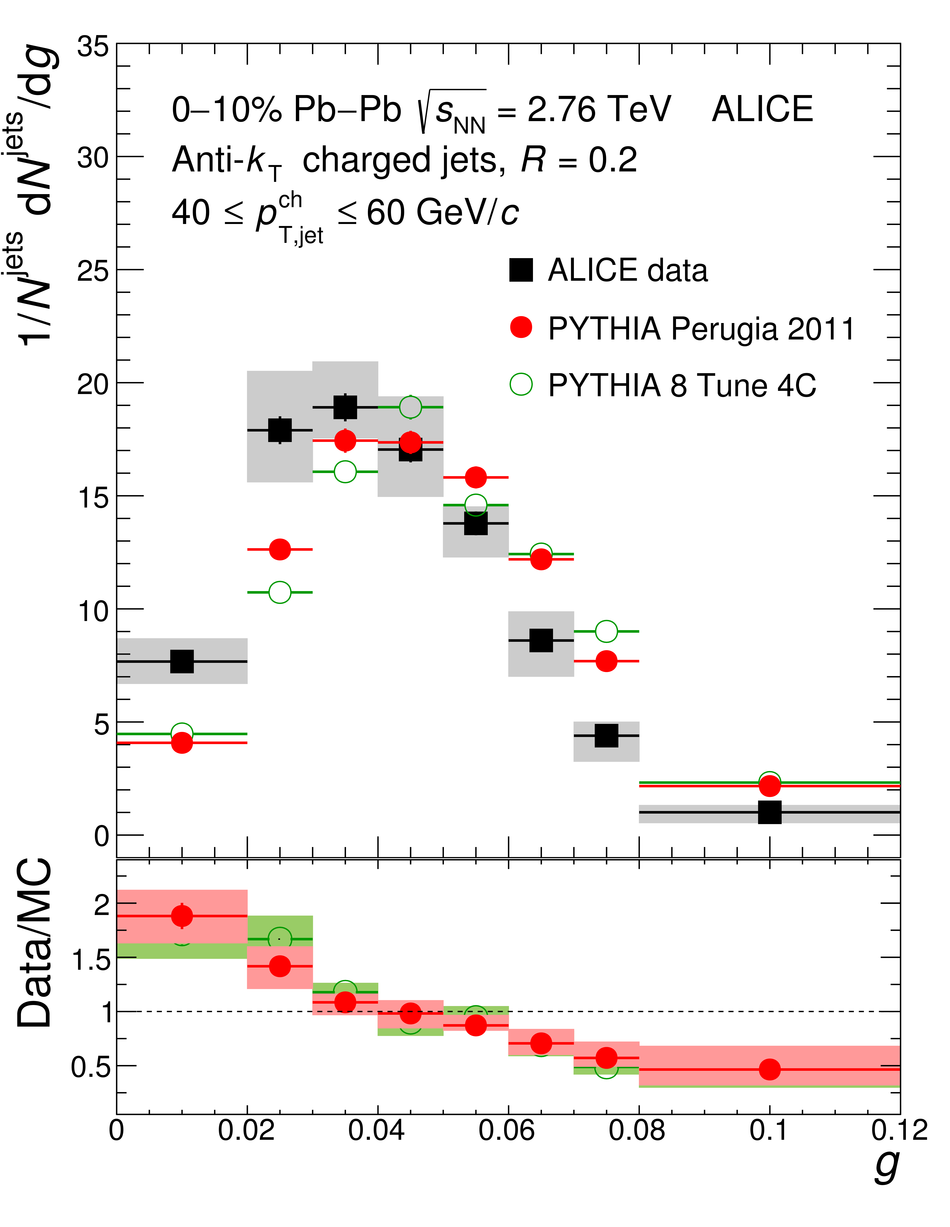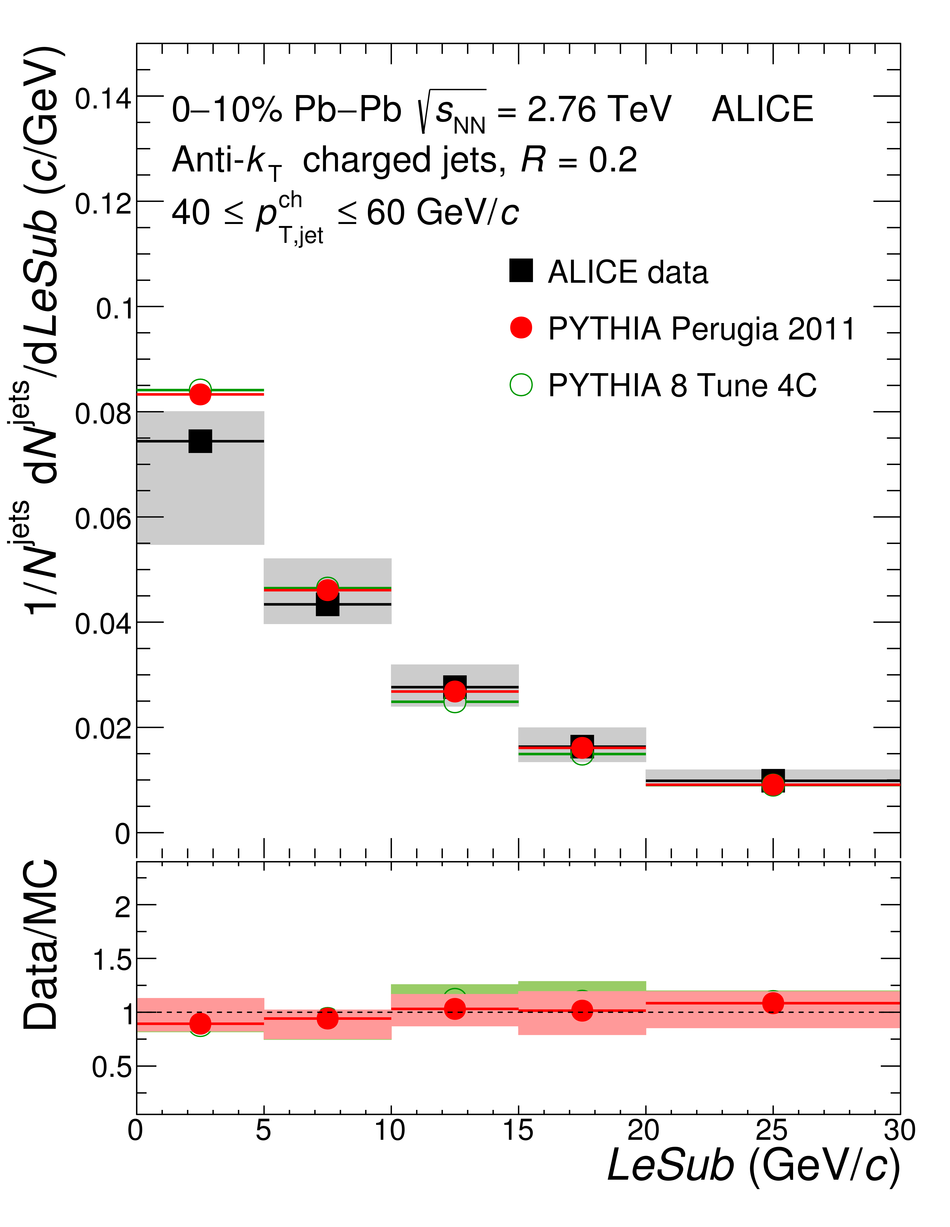# Figure 6

 Fully corrected jet shape distributions in $0$--$10\%$ central Pb--Pb collisions at $\sqrt{s_{\rm NN}} = 2.76$\,TeV for $R = 0.2$ in the range of jet $p_{\mathrm{T,jet}}^{\rm ch}$ of $40$--$60$\,GeV$/c$. The results are compared to PYTHIA. The coloured boxes represent the uncertainty on the jet shape (upper panels) and its propagation to the ratio (lower panels).